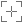# EPSG:6947

## TM75 to WGS 84 (4)

### Attributes

Geodetic CRS: TM75

Datum: Geodetic Datum of 1965

Ellipsoid: Airy Modified 1849

Prime meridian: Greenwich

Data source: EPSG

Information source: OGP

Revision date: 2021-09-23

Scope: (null/copy) Approximation for medium and low accuracy applications assuming equality between plate-fixed static and earth-fixed dynamic CRSs, ignoring static/dynamic CRS differences.

Remarks: Parameter values taken from TM75 to ETRS89 (3) (tfm code 6946) assuming that ETRS89 is coincident with WGS 84 within the accuracy of the tfm. Within accuracy of the tfm equivalent to TM75 to WGS 84 (1) (tfm code 1042).

Method: NTv2

Description: (0,)Ireland - onshore. United Kingdom (UK) - Northern Ireland (Ulster) - onshore.

#### Export

Definition: PROJ.4

`+proj=pipeline +step +proj=axisswap +order=2,1 +step +proj=unitconvert +xy_in=deg +xy_out=rad +step +proj=hgridshift +grids=tm75_etrs89.gsb +step +proj=unitconvert +xy_in=rad +xy_out=deg +step +proj=axisswap +order=2,1`

Definition: JavaScript (Proj4js)

`proj4.defs("EPSG:6947","+proj=pipeline +step +proj=axisswap +order=2,1 +step +proj=unitconvert +xy_in=deg +xy_out=rad +step +proj=hgridshift +grids=tm75_etrs89.gsb +step +proj=unitconvert +xy_in=rad +xy_out=deg +step +proj=axisswap +order=2,1");`

Definition: JSON

```{
"\$schema": "https://proj.org/schemas/v0.4/projjson.schema.json",
"type": "Transformation",
"name": "TM75 to WGS 84 (4)",
"source_crs": {
"type": "GeographicCRS",
"name": "TM75",
"datum": {
"type": "GeodeticReferenceFrame",
"name": "Geodetic Datum of 1965",
"ellipsoid": {
"name": "Airy Modified 1849",
"semi_major_axis": 6377340.189,
"inverse_flattening": 299.3249646
}
},
"coordinate_system": {
"subtype": "ellipsoidal",
"axis": [
{
"name": "Geodetic latitude",
"abbreviation": "Lat",
"direction": "north",
"unit": "degree"
},
{
"name": "Geodetic longitude",
"abbreviation": "Lon",
"direction": "east",
"unit": "degree"
}
]
},
"id": {
"authority": "EPSG",
"code": 4300
}
},
"target_crs": {
"type": "GeographicCRS",
"name": "WGS 84",
"datum_ensemble": {
"name": "World Geodetic System 1984 ensemble",
"members": [
{
"name": "World Geodetic System 1984 (Transit)",
"id": {
"authority": "EPSG",
"code": 1166
}
},
{
"name": "World Geodetic System 1984 (G730)",
"id": {
"authority": "EPSG",
"code": 1152
}
},
{
"name": "World Geodetic System 1984 (G873)",
"id": {
"authority": "EPSG",
"code": 1153
}
},
{
"name": "World Geodetic System 1984 (G1150)",
"id": {
"authority": "EPSG",
"code": 1154
}
},
{
"name": "World Geodetic System 1984 (G1674)",
"id": {
"authority": "EPSG",
"code": 1155
}
},
{
"name": "World Geodetic System 1984 (G1762)",
"id": {
"authority": "EPSG",
"code": 1156
}
},
{
"name": "World Geodetic System 1984 (G2139)",
"id": {
"authority": "EPSG",
"code": 1309
}
}
],
"ellipsoid": {
"name": "WGS 84",
"semi_major_axis": 6378137,
"inverse_flattening": 298.257223563
},
"accuracy": "2.0",
"id": {
"authority": "EPSG",
"code": 6326
}
},
"coordinate_system": {
"subtype": "ellipsoidal",
"axis": [
{
"name": "Geodetic latitude",
"abbreviation": "Lat",
"direction": "north",
"unit": "degree"
},
{
"name": "Geodetic longitude",
"abbreviation": "Lon",
"direction": "east",
"unit": "degree"
}
]
},
"id": {
"authority": "EPSG",
"code": 4326
}
},
"method": {
"name": "NTv2",
"id": {
"authority": "EPSG",
"code": 9615
}
},
"parameters": [
{
"name": "Latitude and longitude difference file",
"value": "tm75_etrs89.gsb",
"id": {
"authority": "EPSG",
"code": 8656
}
}
],
"accuracy": "1.0",
"scope": "(null/copy) Approximation for medium and low accuracy applications assuming equality between plate-fixed static and earth-fixed dynamic CRSs, ignoring static/dynamic CRS differences.",
"area": "Ireland - onshore. United Kingdom (UK) - Northern Ireland (Ulster) - onshore.",
"bbox": {
"south_latitude": 51.39,
"west_longitude": -10.56,
"north_latitude": 55.43,
"east_longitude": -5.34
},
"id": {
"authority": "EPSG",
"code": 6947
},
"remarks": "Parameter values taken from TM75 to ETRS89 (3) (tfm code 6946) assuming that ETRS89 is coincident with WGS 84 within the accuracy of the tfm. Within accuracy of the tfm equivalent to TM75 to WGS 84 (1) (tfm code 1042)."
}
```

Definition: MapServer - MAPfile

```PROJECTION
"proj=pipeline"
"step"
"proj=axisswap"
"order=2,1"
"step"
"proj=unitconvert"
"xy_in=deg"
"step"
"proj=hgridshift"
"grids=tm75_etrs89.gsb"
"step"
"proj=unitconvert"
"xy_out=deg"
"step"
"proj=axisswap"
"order=2,1"
END```

Definition: Mapnik

```<?xml version="1.0" encoding="utf-8"?>
<Map srs="+proj=pipeline +step +proj=axisswap +order=2,1 +step +proj=unitconvert +xy_in=deg +xy_out=rad +step +proj=hgridshift +grids=tm75_etrs89.gsb +step +proj=unitconvert +xy_in=rad +xy_out=deg +step +proj=axisswap +order=2,1">
<Layer srs="+proj=pipeline +step +proj=axisswap +order=2,1 +step +proj=unitconvert +xy_in=deg +xy_out=rad +step +proj=hgridshift +grids=tm75_etrs89.gsb +step +proj=unitconvert +xy_in=rad +xy_out=deg +step +proj=axisswap +order=2,1">
</Layer>
</Map>```

Definition: SQL (PostGIS)

`INSERT into spatial_ref_sys (srid, auth_name, auth_srid, proj4text, srtext) values ( 6947, 'EPSG', 6947, '+proj=pipeline +step +proj=axisswap +order=2,1 +step +proj=unitconvert +xy_in=deg +xy_out=rad +step +proj=hgridshift +grids=tm75_etrs89.gsb +step +proj=unitconvert +xy_in=rad +xy_out=deg +step +proj=axisswap +order=2,1', 'None');`

Definition: OGC WKT 2 (2019)

`COORDINATEOPERATION["TM75 to WGS 84 (4)",VERSION["OGP-Ire NT"],SOURCECRS[GEOGCRS["TM75",DATUM["Geodetic Datum of 1965",ELLIPSOID["Airy Modified 1849",6377340.189,299.3249646,LENGTHUNIT["metre",1]]],PRIMEM["Greenwich",0,ANGLEUNIT["degree",0.0174532925199433]],CS[ellipsoidal,2],AXIS["geodetic latitude (Lat)",north,ORDER,ANGLEUNIT["degree",0.0174532925199433]],AXIS["geodetic longitude (Lon)",east,ORDER,ANGLEUNIT["degree",0.0174532925199433]],ID["EPSG",4300]]],TARGETCRS[GEOGCRS["WGS 84",ENSEMBLE["World Geodetic System 1984 ensemble",MEMBER["World Geodetic System 1984 (Transit)"],MEMBER["World Geodetic System 1984 (G730)"],MEMBER["World Geodetic System 1984 (G873)"],MEMBER["World Geodetic System 1984 (G1150)"],MEMBER["World Geodetic System 1984 (G1674)"],MEMBER["World Geodetic System 1984 (G1762)"],MEMBER["World Geodetic System 1984 (G2139)"],ELLIPSOID["WGS 84",6378137,298.257223563,LENGTHUNIT["metre",1]],ENSEMBLEACCURACY[2.0]],PRIMEM["Greenwich",0,ANGLEUNIT["degree",0.0174532925199433]],CS[ellipsoidal,2],AXIS["geodetic latitude (Lat)",north,ORDER,ANGLEUNIT["degree",0.0174532925199433]],AXIS["geodetic longitude (Lon)",east,ORDER,ANGLEUNIT["degree",0.0174532925199433]],ID["EPSG",4326]]],METHOD["NTv2",ID["EPSG",9615]],PARAMETERFILE["Latitude and longitude difference file","tm75_etrs89.gsb"],OPERATIONACCURACY[1.0],USAGE[SCOPE["(null/copy) Approximation for medium and low accuracy applications assuming equality between plate-fixed static and earth-fixed dynamic CRSs, ignoring static/dynamic CRS differences."],AREA["Ireland - onshore. United Kingdom (UK) - Northern Ireland (Ulster) - onshore."],BBOX[51.39,-10.56,55.43,-5.34]],ID["EPSG",6947],REMARK["Parameter values taken from TM75 to ETRS89 (3) (tfm code 6946) assuming that ETRS89 is coincident with WGS 84 within the accuracy of the tfm. Within accuracy of the tfm equivalent to TM75 to WGS 84 (1) (tfm code 1042)."]]`